# Metallurgical Engineering - MT 2012 GATE Paper (Practice Test)

## 65 Questions MCQ Test GATE Past Year Papers for Practice (All Branches) | Metallurgical Engineering - MT 2012 GATE Paper (Practice Test)

Description
Attempt Metallurgical Engineering - MT 2012 GATE Paper (Practice Test) | 65 questions in 180 minutes | Mock test for GATE preparation | Free important questions MCQ to study GATE Past Year Papers for Practice (All Branches) for GATE Exam | Download free PDF with solutions
QUESTION: 1

Solution:
QUESTION: 2

Solution:
QUESTION: 3

### Choose the most appropriate alternative from the options given below to complete the following sentence: If the tired soldier wanted to lie down, he ___ the mattress out on the balcony.

Solution:
QUESTION: 4

If (1.001)1259 = 3.52 and (1.001)2062 = 7.85, then (1.001)3321 =

Solution:
QUESTION: 5

One of the parts (A, B, C, D) in the sentence given below contains an ERROR. Which one of the following is INCORRECT?
I requested that he should be given the driving test today instead of tomorrow.

Solution:
QUESTION: 6

Q. 6 - Q. 10 carry two marks each.
Q. The data given in the following table summarizes the monthly budget of an average household.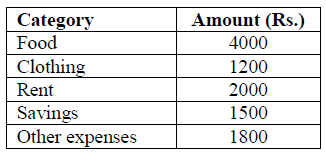The approximate percentage of the monthly budget NOT spent on savings is

Solution:
QUESTION: 7

There are eight bags of rice looking alike, seven of which have equal weight and one is slightly heavier. The weighing balance is of unlimited capacity. Using this balance, the minimum number of weighings required to identify the heavier bag is

Solution:
QUESTION: 8

Raju has 14 currency notes in his pocket consisting of only Rs. 20 notes and Rs. 10 notes. The total money value of the notes is Rs. 230. The number of Rs. 10 notes that Raju has is

Solution:
QUESTION: 9

A and B are friends. They decide to meet between 1 PM and 2 PM on a given day. There is a condition that whoever arrives first will not wait for the other for more than 15 minutes. The probability that they will meet on that day is

Solution:
QUESTION: 10

One of the legacies of the Roman legions was discipline. In the legions, military law prevailed and discipline was brutal. Discipline on the battlefield kept units obedient, intact and fighting, even when the odds and conditions were against them.

Which one of the following statements best sums up the meaning of the above passage?

Solution:
QUESTION: 11

Useful Data
Universal gas constant R = 8.314 J/mol.K
Planck’s constant h = 6.63*10-34 J.s
Acceleration due to gravity g = 9.8 m/s2

Q. 11 – Q. 35 carry one mark each.

A is a 2 * 2 matrix with det A = 2. The det (2A) is

Solution:
QUESTION: 12

A is a 2 * 2 matrix given below: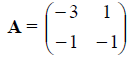The eigenvalues of A are

Solution:
QUESTION: 13

In a production facility, iron rods are made with a mean diameter of 6 cm and standard deviation of 0.02 cm. If a large number of rods are tested, the approximate percentage of rods whose sizes fall in the range of 5.98 cm to 6.02 cm is

Solution:
QUESTION: 14

Which one of the following methods is NOT used for numerical integration?

Solution:
QUESTION: 15

How many boundary conditions are required to solve the following equation?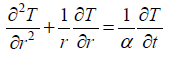Solution:
QUESTION: 16

When a zinc metal rod is immersed in dilute hydrochloric acid, it results in

Solution:
QUESTION: 17

A fluid is flowing with a velocity of 0.5 m/s on a plate moving with a velocity of 0.01 m/s in the same direction. The velocity at the interface of the fluid and plate is

Solution:
QUESTION: 18

Hot metal at 1700 K is poured in a sand mould that is open at the top. Heat loss from the liquid metal takes place by

Solution:
QUESTION: 19

Which one of the following is an equilibrium defect?

Solution:
QUESTION: 20

Floatation beneficiation is based on the principle of

Solution:
QUESTION: 21

Copper can be reduced from acidic copper sulphate solution by

Solution:
QUESTION: 22

Which one is NOT an agglomeration process?

Solution:
QUESTION: 23

During LD blow in steelmaking the impurity that gets removed first is

Solution:
QUESTION: 24

During the solidification of a pure metal, it was found that dendrites are formed. Assuming that the liquid-solid interface is at the melting temperature, the temperature from the interface into the liquid

Solution:
QUESTION: 25

A peak in the X-ray diffraction pattern is observed at 2θ = 78°, corresponding to {311} planes of an fcc metal, when the incident beam has a wavelength of 0.154 nm. The lattice parameter of the metal is approximately

Solution:
QUESTION: 26

If d is the inter-planar spacing of the planes {h k l}, the inter-planar spacing of the planes {nh nk nl}, n being an integer, is

Solution:
QUESTION: 27

As temperature increases, the electrical resistivities of pure metals (ρm) and intrinsic semiconductors (ρs) vary as follows

Solution:
QUESTION: 28

At equilibrium spacing in a crystalline solid, which of the following is true for net inter-atomic force (F) and potential energy (U)

Solution:
QUESTION: 29

The property of a material that CANNOT be significantly changed by heat treatment is

Solution:
QUESTION: 30

A unit dislocation splits into two partial dislocations. The correct combination of the Burgers vectors of the partial dislocations for a given unit dislocation having Burgers vector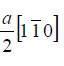Solution:
QUESTION: 31

A polymer matrix composite is reinforced with long continuous ceramic fibres aligned in one direction. The Young’s moduli of the matrix and fibres are Em and Ef respectively, and the volume fraction of the fibres is f. Assuming iso-stress condition, Young’s modulus of the composite EC in a direction perpendicular to the length of fibres, is given by the expression

Solution:
QUESTION: 32

Which of the following is NOT a fusion welding process?

Solution:
QUESTION: 33

Tungsten filament used in electric bulb is processed by

Solution:
QUESTION: 34

The riser is designed such that the melt in the riser solidifies

Solution:
QUESTION: 35

Radiography technique of detecting defects is based on the principle of

Solution:
QUESTION: 36

Q. 36 to Q. 65 carry two marks each.

At x = 0.5, the polynomial x2(1-x)2 has

Solution:
QUESTION: 37

Given that v is a vector field and f is a scalar field, match the equations in Group I with their physical meaning in Group II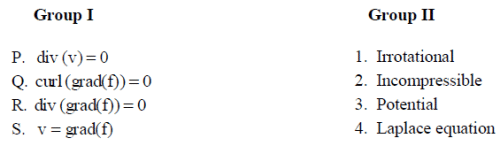Solution:
QUESTION: 38

The temperature field of a slab is given by T = 400 – 50z exp (–t – x2 – y2). The temperature gradient in y-direction is

Solution:
QUESTION: 39

What does the solution of the following ordinary differential equation represent?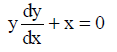Solution:
QUESTION: 40

A thin layer of material B (of total amount m) is plated on the end faces of two long rods of material A. These are then joined together on the plated side (see the figure below) and heated to a high temperature. Assuming the diffusion coefficient of B in A is D, the composition profile cB along the rod axis x after a time t is described by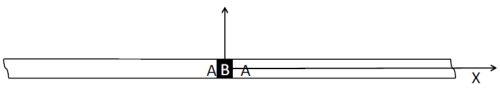Solution:
QUESTION: 41

Match the principles given in Group I with corresponding corrosion terminology in Group II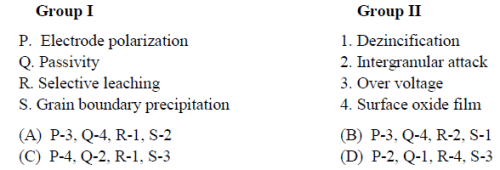Solution:
QUESTION: 42

Identify the correct combination of the following statements

P. Hydrogen electrode is a standard used to measure redox potentials
Q. Activation polarization refers to electrochemical processes controlled by reaction sequence at metal-solution interface
R. Potential-pH diagrams can be used to predict corrosion rates of metals
S. Cathodic protection can use sacrificial anodes such as magnesium

Solution:
QUESTION: 43

Consider a reaction with activation energy of 8.314 kJ/mol that takes place at 300 K. If the reaction rate is to be tripled, the temperature of the reaction should be

Solution:
QUESTION: 44

Match the processes in Group I with the objectives in Group II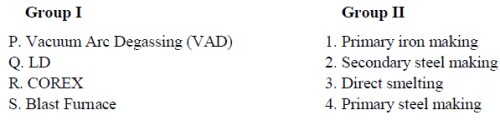Solution:
QUESTION: 45

The reduction of FeO with CO gas in co-current flow is given by the following equation: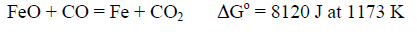The ratio of pCO/pCO2 for this reaction at 1173 K is

Solution:
QUESTION: 46

The sulphide capacity (CS) of liquid slag of composition 55 wt.% CaO, 20 wt.% SiO2, 15 wt.% Al2O3, and 10 wt.% MgO is given by the following equation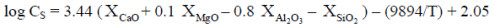where, X is mole fraction of the respective components. Atomic weights of Ca, Mg, Si, Al and O are 40, 24, 28, 27 and 16 respectively.

The value of CS at 1900 K is

Solution:
QUESTION: 47

Match the processes given in Group I with the corresponding metals in Group II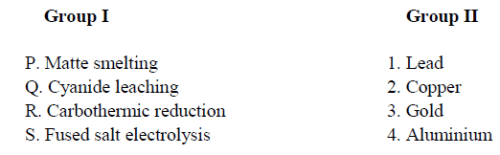Solution:
QUESTION: 48

Identify the correct combination of the following statements

P. Bessemer converter can be used in copper smelting
Q. The Mond process for nickel involves reaction of metal with H2 gas
R. Roasted ZnS concentrates can be smelted in a blast furnace
S. Magnesium metal can be produced by electrolysis of sea water

Solution:
QUESTION: 49

Match the phases of steel in Group I with the crystal structures in Group II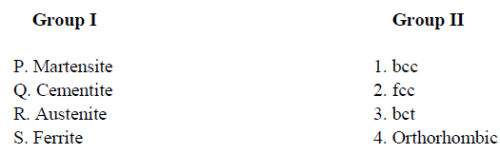Solution:
QUESTION: 50

Arrange the following in terms of increasing severity of quench

P. Oil quenching
Q. Water quenching
R. Water quenching with agitation
S. Brine quenching

Solution:
QUESTION: 51

Regarding recrystallization, which one of the following statements is NOT correct?

Solution:
QUESTION: 52

A liquid droplet (β) is on a substrate (δ) and is surrounded by air (α), as shown below. The angle of contact (θ) is determined using the following expression: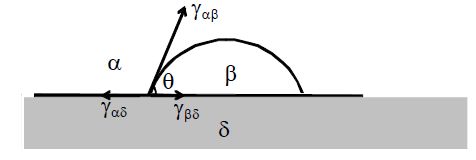Solution:
QUESTION: 53

Match the phenomena listed in Group I with the possible mechanisms in Group II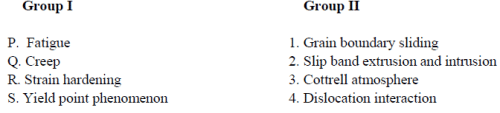Solution:
QUESTION: 54

Fracture stress for a brittle material having a crack length of 1 μm is 200 MPa. Fracture stress for the same material having a crack length of 4 μm is

Solution:
QUESTION: 55

The flow stress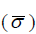of an alloy varies with strain rate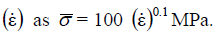When the alloy is hot extruded from 10 cm diameter to 5 cm diameter at a speed of 2 cm/s, the flow stress is

Solution:
QUESTION: 56

Determine the correctness or otherwise of the following Assertion (a) and Reason (r).

Assertion : During rolling, front tension and (or) back tension are (is) employed to decrease rolling load.
Reason : Roll pressure decreases due to lowering of flow stress as a result of front tension/back tension.

Solution:
QUESTION: 57

Match the defects listed in Group I with the processes listed in Group II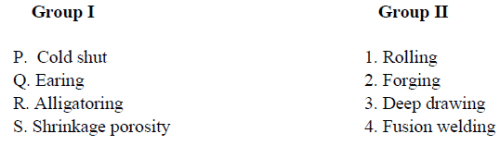Solution:
QUESTION: 58

Common Data Questions

Common Data for Questions 58 and 59:

A steel ball (density ρsteel = 7200 kg/m3) is placed in an upward moving liquid Al (density ρAl = 2360 kg/m3,
viscosity μAl = 1*10–3 Pa.s and Reynolds number = 5*105). The force (F) exerted on the steel ball is
expressed as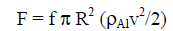where, f is friction factor (=0.2), v is the velocity of liquid Al and R is the radius of steel ball.

The force exerted on the steel ball is

Solution:
QUESTION: 59

A steel ball (density ρsteel = 7200 kg/m3) is placed in an upward moving liquid Al (density ρAl = 2360 kg/m3,
viscosity μAl = 1*10–3 Pa.s and Reynolds number = 5*105). The force (F) exerted on the steel ball is
expressed aswhere, f is friction factor (=0.2), v is the velocity of liquid Al and R is the radius of steel ball.

The terminal velocity of a fine spherical steel particle having diameter dp, in μm range, if allowed to fall in a quiescent liquid Al bath, is

Solution:
QUESTION: 60

Common Data for Questions 60 and 61: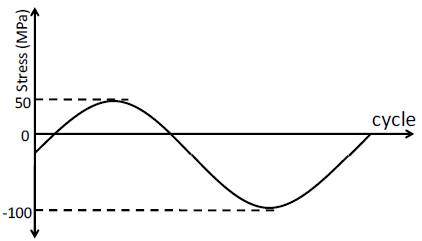For the above stress cycle

Stress ratio is

Solution:
QUESTION: 61For the above stress cycle

Amplitude ratio is

Solution:
QUESTION: 62

A material with grain size of ASTM No. 6 has a lattice frictional stress 100 MN/m2 and locking parameter
(Hall-Petch constant) 0.10 MN/m3/2

Grain size of the material is approximately

Solution:
QUESTION: 63

A material with grain size of ASTM No. 6 has a lattice frictional stress 100 MN/m2 and locking parameter
(Hall-Petch constant) 0.10 MN/m3/2

Yield strength of the material is approximately

Solution:
QUESTION: 64

The strain hardening behaviour of an annealed rod during cold rolling is given by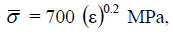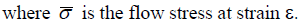Flow stress after 50% reduction in area of the annealed rod on cold rolling is approximately

Solution:
QUESTION: 65

The strain hardening behaviour of an annealed rod during cold rolling is given byIf a wire of 5 mm diameter is drawn from the above cold rolled rod of 10 mm diameter, the drawingstress, neglecting the effect of friction and redundant work, is approximately

Solution:Use Code STAYHOME200 and get INR 200 additional OFF Use Coupon Code